# 程序控制结构

## 选择结构

if 结构：if (表达式){}  表达式为真则执行，不为真则不执行

if-else 结构： if中表达式为真则执行if表达式后接的{}中内容，不为真则执行else{}中内容

if-elseif-else结构：f中表达式为真则执行if表达式后接的{}中内容，不为真继续向下判断下一个elseif,为真则执行elseif{}中内容，前面的都不满足，执行最后一个else{}中内容。

``````#include <iostream>
using namespace std;
int main()
{
// 选择结构 if语句
// 场景: 定义一个整型表示上班打卡时间，超过9点打卡视为迟到,9点前为未迟到。
int time = 0;
cout << "Please enter the clock in time:" << endl;
cin >> time; //  第一次输入10  第二次输入8
if (time > 9)   // 如果里面的表达式time > 9 返回真则执行他下面的{}中内容，如果为假则执行else后{}中内容
{
cout << "You're late!" << endl; // 第一次输出 You're late!
}else{
cout << "You're not late!" << endl; //第二次输出 You're not late!"
}
system("pause");
return 0;
}``````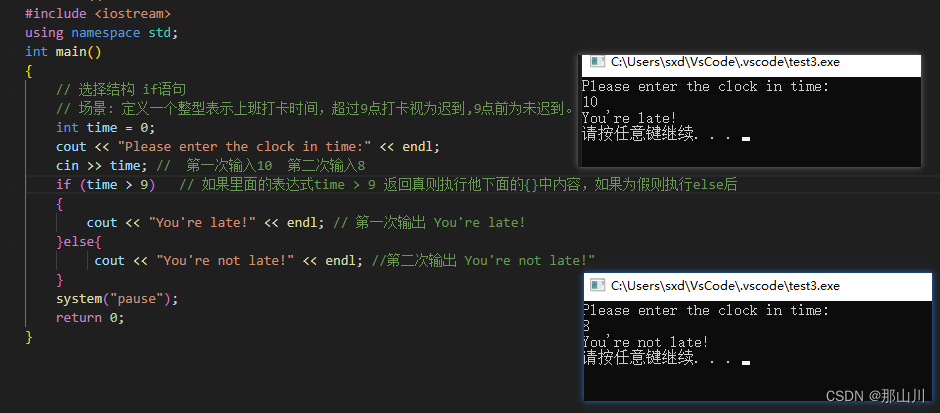## 循环结构

### while循环

``````#include <iostream>
using namespace std;
int main()
{
// while 循环输出0-9
int i = 0;
while (i < 10)   // 输出9 表达式需要小于10 或者<=9
{
cout << i << endl;
i++; // 进行+1操作然后判断i < 10 程序需要可中止，不能陷入死循环
}
system("pause");
}``````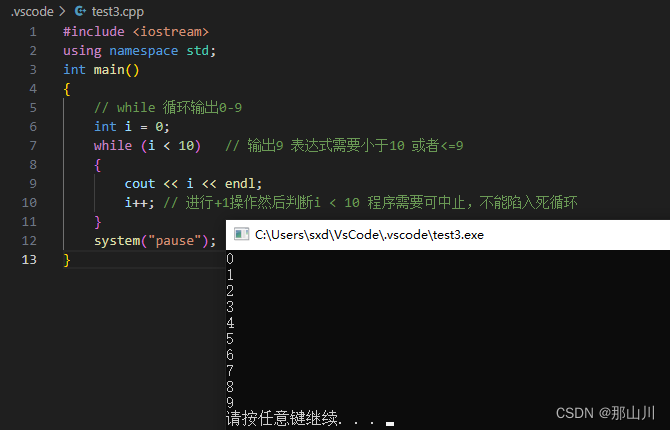### do...while循环

``````#include <iostream>
using namespace std;
int main()
{
// while 循环输出0-9
int i = 0;
do
{
cout << i << endl;
i++; // 进行+1操作然后判断i < 10 程序需要可中止，不能陷入死循环
}  while (i< 10); // 正常输出0-10
// 如果上面的条件换成while (i > 10); 那么显然i=0不满足，但是do...while是先执行一次do中内容 所以控制台还是会输出 0
system("pause");
}``````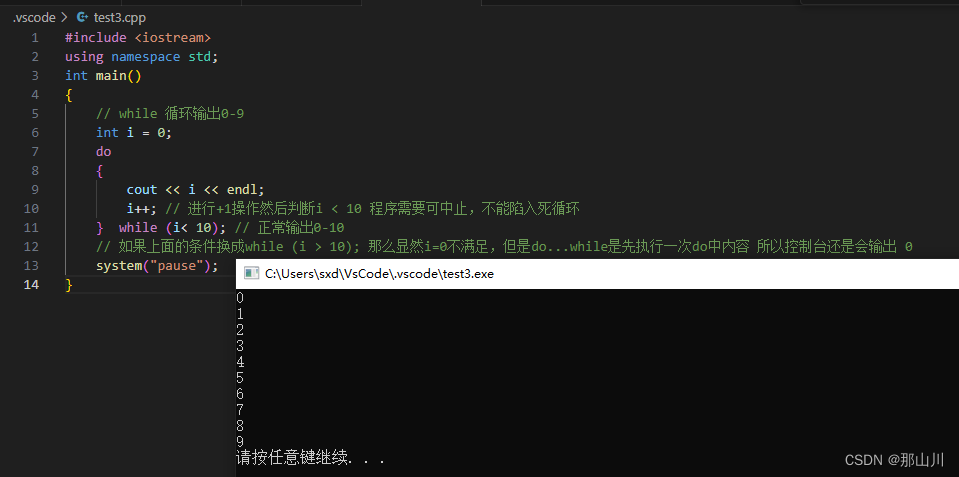``````#include <iostream>
using namespace std;
int main()
{
int i = 0;
do
{
cout << i << endl;
i++;
}  while (i);
system("pause");
}``````

#### 案例：水仙花

``````#include <iostream>
using namespace std;
int main()
{
// 什么是水仙花数？
// 水仙花数是指一个 n 位数（n ≥ 3），它的每个位上的数字的 n 次幂之和等于它本身。例如，153 是一个水仙花数，因为 1^3 + 5^3 + 3^3 = 153。
// 换句话说，一个三位数的水仙花数是指满足以下条件的数字：
// 如果将该数字的个位、十位和百位分别记为 a、b 和 c，则满足 a^3 + b^3 + c^3 = abc。
int num = 100; // 100-999 水仙花数
do
{
int j = 0;         // 个位数字
int k = 0;         // 十位数字
int l = 0;         // 百位数字
j = num % 10;      // 获取个位数字
k = num / 10 % 10; // 获取十位数字
l = num / 100;     // 获取百位数字
// 找水仙花数
if (num == j * j * j + k * k * k + l * l * l)   // 这里判断是== 切记
{
cout <<num<<endl;
}
num++;
} while (num < 1000);

system("pause");
}``````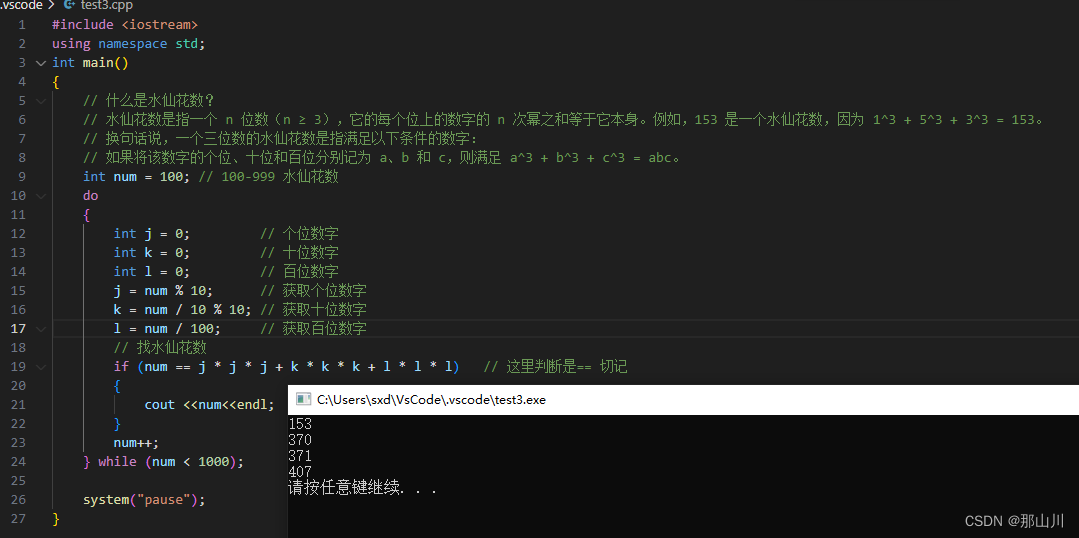### for循环语句

``````#include <iostream>
using namespace std;
int main()
{
// for循环 语法：for(起始表达式;条件表达式;末尾循环体){循环语句;}
// 从0打印到数字9
for (int i = 0; i < 10; i++) // 执行顺序 ①int i= 0；②i<10 ③ cout << i << endl; ④i++ 然后继续循环 ②i<10 ③ cout << i << endl; ④i++直到 ②i<10已经不满足就退出了循环
{
cout << i << endl;
}
system("pause");
}``````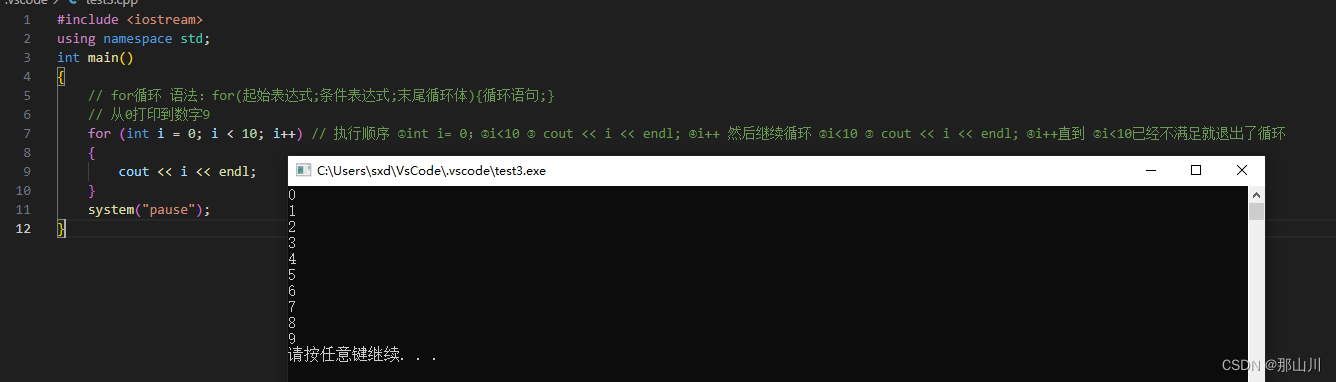### 嵌套循环

#### 案例：九九乘法表

``````#include <iostream>
using namespace std;
int main()
// 九九乘法表 列数*行数 = 计算结果
{
for (int i = 1; i <= 9; i++)
{
for (int j = 1; j <= i; j++)
{
cout << j << " * " << i << " = " << j * i<<"\t"; // \t转义字符 增加个缩进，更加美观
}
cout << endl;
}

system("pause");
}``````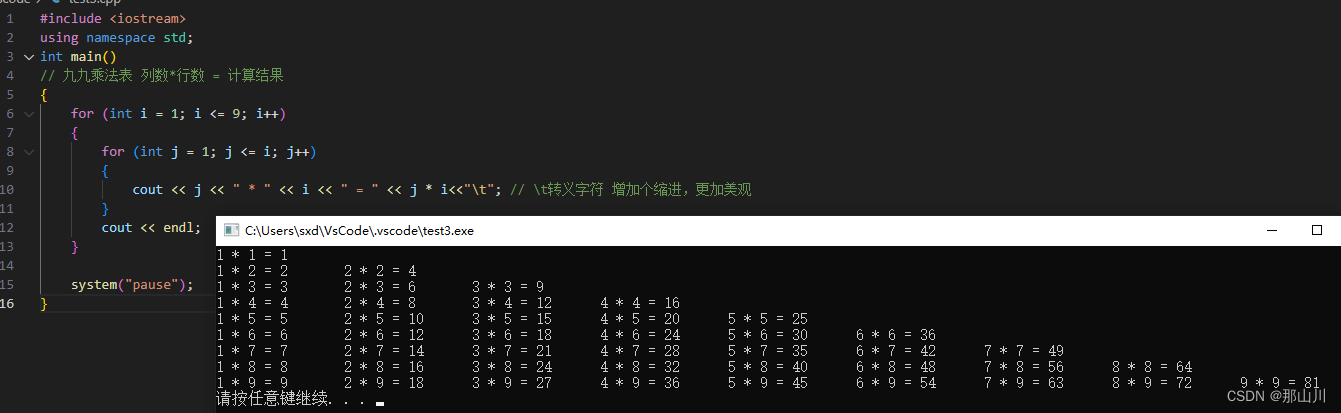## 三目运算符

``````#include <iostream>
using namespace std;
int main()
{
// 需求 比较a、b、c中最大的数 如果这个你可以看懂 你的三目运算应该问题不大了
int a = 5;
int b = 10;
int c = 17;
// 拆解
// 1.首先判断(a>b)?a:b 找出最大的值
// 由于我们一条写完 就直接拿他和c比较，如果c大输出c，否则输出(a>b)?a:b ->a、b中的更大的值。
cout<< (((a>b)?a:b)>c?((a>b)?a:b):c);
system("pause");
return 0;
}``````

## switch语句

switch(表达式)

{

case 结果1:  执行语句;break;

case 结果2:  执行语句;break;

...

default: 执行语句;break;

}

``````#include <iostream>
using namespace std;
int main()
{
// 给电影评分 10~9 非常好； 8~7 好 ；6~5 一般； <5 差；
int score=0;
// 请输入评价分
cout<<"Please enter the score for the evaluation"<<endl;
cin>>score;
switch (score)
{
case 10:
case 9:
cout<<"very good"<<endl;
break;
case 8:
case 7:
cout<<"good"<<endl;
break;
case 6:
case 5:
cout<<"normal"<<endl;
break;
default:
break;
}
system("pause");
return 0;
}``````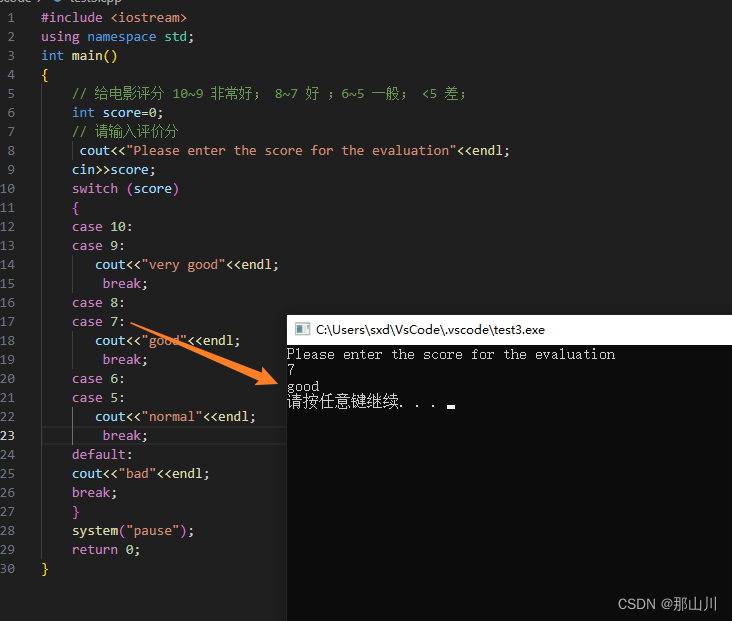swiitch语句中表达式类型是整型或字符型

case中没有break，程序会继续向下执行，不终止。

## 跳转语句

### break语句

1. switch语句中，作用为终止case并跳出switch语句。

2. 循环语句中，跳出当前的循环语句。

3. 嵌套循环语句中，跳出最近的内层循环语句。

``````#include <iostream>
using namespace std;
int main()
// break

// 1. switch语句中，作用为终止case并跳出switch语句。
{
// // 给电影评分 10~9 非常好； 8~7 好 ；6~5 一般； <5 差；
// int score=0;
// // 请输入评价分
//  cout<<"Please enter the score for the evaluation"<<endl;
// cin>>score;
// switch (score)
// {
// case 10:
// case 9:
//    cout<<"very good"<<endl;
//     break;
// case 8:
// case 7:
//    cout<<"good"<<endl;
//     break;
// case 6:
// case 5:
//    cout<<"normal"<<endl;
//     break;
// default:
// break;
// }
//----------------------------------------------------------------------------//

// 2. 循环语句中，跳出当前的循环语句。
// // 输入数字，输入为5时退出,最多输入100次

// for (int i = 0; i < 100; i++)
// {
//     cout << "Enter a num" << endl;
//     cin >> i;
//     if (i == 5)
//     {
//         cout << "ok" << endl;
//         break;
//     }
// }
//----------------------------------------------------------------------------//

// 3. 嵌套循环语句中，跳出最近的内层循环语句。
for (int i = 0; i < 10; i++)
{
for (int j = 0; j < 10; j++)
{
if (j >= 5)
{
break; // 正常情况下我们是画了一个10行10列的矩形* 现在加了判断 内层次j>=5就会跳出内层for循环开始i=1的内层循环遍历。
// 最终结果就会打印出一个5列10行的矩形*
}

cout << "*";
}
cout << endl;
}

system("pause");
return 0;
}``````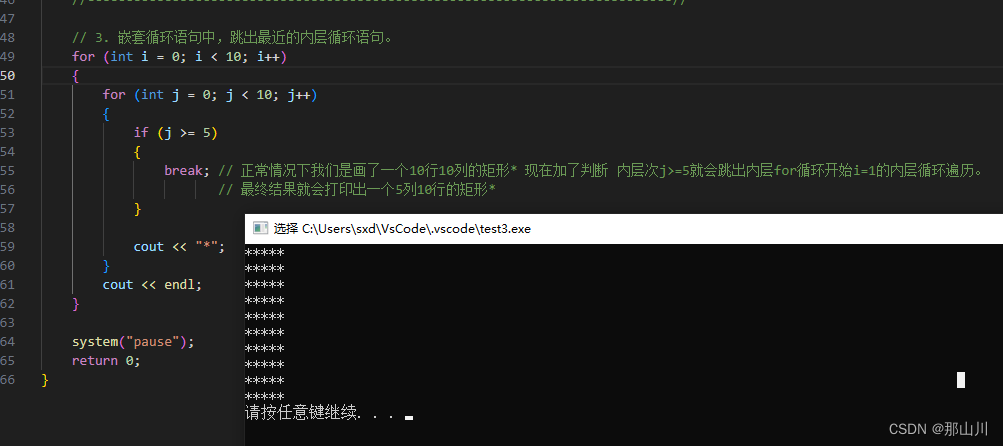### continue

continue 跳出本次循环

break与continue的区别：

• `break` 用于完全终止循环，跳出循环体。
• `continue` 用于跳过当前迭代的剩余代码，开始下一次迭代。
``````#include <iostream>
using namespace std;
int main()
{
// continue
//  // 打印 1 到 10 之间的奇数
for (int i = 0; i <= 10; i++)
{
if (i % 2 == 0) // 为偶数直接跳过本次for 开始下一次循环
{
continue;
}
cout << i << endl;
}
system("pause");
return 0;
}
``````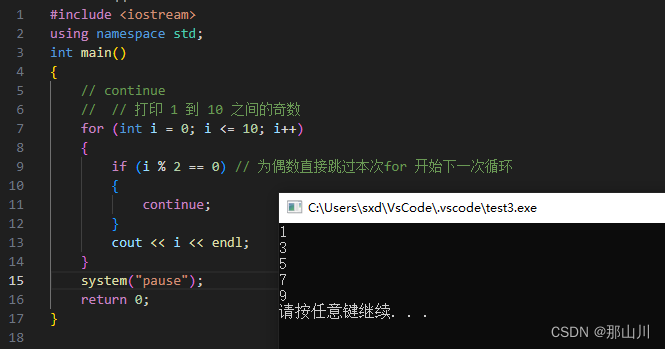### goto

``````#include <iostream>
using namespace std;
int main()
{
// goto
cout<<"1"<<endl;
goto flag; // 跳转到标记位置
cout<<"2"<<endl;
flag:    // 标记在此位置
cout<<"3"<<endl;
cout<<"4"<<endl;
system("pause");
return 0;
}
``````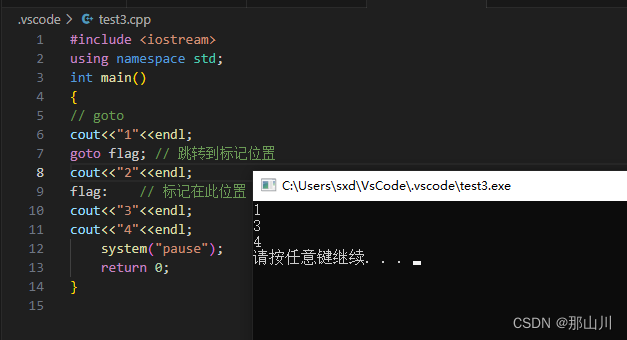注意：在程序中尽量不要使用goto语句，以免造成程序流程的混乱。